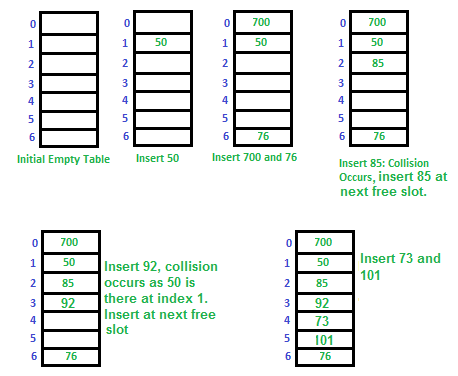# Hashing | Set 3 (Open Addressing)

We strongly recommend to refer below post as a prerequisite of this.
Hashing | Set 1 (Introduction)
Hashing | Set 2 (Separate Chaining)

Like separate chaining, open addressing is a method for handling collisions. In Open Addressing, all elements are stored in the hash table itself. So at any point, size of the table must be greater than or equal to the total number of keys (Note that we can increase table size by copying old data if needed).

Insert(k): Keep probing until an empty slot is found. Once an empty slot is found, insert k.

Search(k): Keep probing until slot’s key doesn’t become equal to k or an empty slot is reached.

Delete(k): Delete operation is interesting. If we simply delete a key, then search may fail. So slots of deleted keys are marked specially as “deleted”.
Insert can insert an item in a deleted slot, but the search doesn’t stop at a deleted slot.

Open Addressing is done following ways:

a) Linear Probing: In linear probing, we linearly probe for next slot. For example, typical gap between two probes is 1 as taken in below example also.
let hash(x) be the slot index computed using hash function and S be the table size

```If slot hash(x) % S is full, then we try (hash(x) + 1) % S
If (hash(x) + 1) % S is also full, then we try (hash(x) + 2) % S
If (hash(x) + 2) % S is also full, then we try (hash(x) + 3) % S
..................................................
..................................................```

Let us consider a simple hash function as “key mod 7” and sequence of keys as 50, 700, 76, 85, 92, 73, 101.Clustering: The main problem with linear probing is clustering, many consecutive elements form groups and it starts taking time to find a free slot or to search an element.

b) Quadratic Probing We look for i2‘th slot in i’th iteration.

```let hash(x) be the slot index computed using hash function.
If slot hash(x) % S is full, then we try (hash(x) + 1*1) % S
If (hash(x) + 1*1) % S is also full, then we try (hash(x) + 2*2) % S
If (hash(x) + 2*2) % S is also full, then we try (hash(x) + 3*3) % S
..................................................
..................................................```

c) Double Hashing We use another hash function hash2(x) and look for i*hash2(x) slot in i’th rotation.

```let hash(x) be the slot index computed using hash function.
If slot hash(x) % S is full, then we try (hash(x) + 1*hash2(x)) % S
If (hash(x) + 1*hash2(x)) % S is also full, then we try (hash(x) + 2*hash2(x)) % S
If (hash(x) + 2*hash2(x)) % S is also full, then we try (hash(x) + 3*hash2(x)) % S
..................................................
..................................................```

See this for step by step diagrams.

Comparison of above three:
Linear probing has the best cache performance but suffers from clustering. One more advantage of Linear probing is easy to compute.

Quadratic probing lies between the two in terms of cache performance and clustering.

Double hashing has poor cache performance but no clustering. Double hashing requires more computation time as two hash functions need to be computed.

Like Chaining, the performance of hashing can be evaluated under the assumption that each key is equally likely to be hashed to any slot of the table (simple uniform hashing)

``` m = Number of slots in the hash table
n = Number of keys to be inserted in the hash table

Load factor α = n/m  ( < 1 )

Expected time to search/insert/delete < 1/(1 - α)

So Search, Insert and Delete take (1/(1 - α)) time
```

Don’t stop now and take your learning to the next level. Learn all the important concepts of Data Structures and Algorithms with the help of the most trusted course: DSA Self Paced. Become industry ready at a student-friendly price.

My Personal Notes arrow_drop_up

Improved By : PulkitGoel2, shubham_singh

Article Tags :
Practice Tags :

55

Please write to us at contribute@geeksforgeeks.org to report any issue with the above content.